Polar of a point with respect to two lines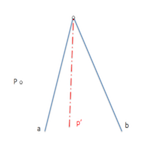The concept of polarity is linked to the harmonic separation.

This concept is Basic for the determination of the fundamental elements of conics, as its Center, conjugate diameters, axes ….

It will allow to establish new transformations which include homographies and correlations of great importance.

We can see different definitions associated with the concepts that we will see below, in this case focusing on the determination of the polar line of a point with respect to two given lines.

We will remember that given four points A, B, C and D, located on a straight line, We can define the double reason of these four points (ABCD) as the ratio of the simple reasons (ACD) and (BCD). The double reason studied it to define the quadruples of items ordered While the simple reason was formulated in the introduction to ordered triples of elements.

We similarly termed the double reason for four-straight, represented as (abcd), and we residual is why double with the points scored when sectioning these straight lines, being equal and therefore (ABCD)=(abcd)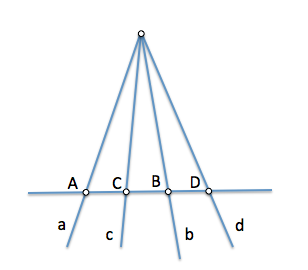When is the value of the double reason “-1”, namely, the negative unit, We say that the elements of the Tetrad (ABCD)=(abcd)=-1 determine a harmonic Tetrad, and as a result the first two elements, points or lines, harmoniously separated both of them late each Tetrad, namely:

• It (ABCD)=-1 then “A” and “B” harmoniously separated to “C” and “D”
• It (abcd)=-1 then “to” and “b” separate harmonically to “c” and “d”

This same text used it to analyze the Harmonic relations in the full cuadrivertice, relationships that will now be very useful for the determination of the polar of a point with respect to two lines.

Be a point P and two lines “to” and “b” It does not contain him.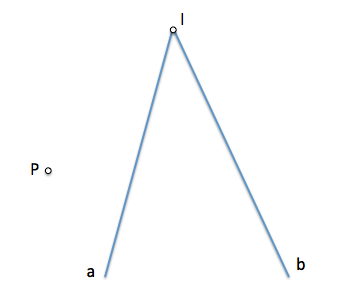We seccionemos to the straight lines “to” and “b” by a straight anyone who passes by “P“. This straight cut at the points “A” and “B” to the previous straight lines. Be the point “P’” a point located between “A” and “B“, so that (PP ’ AB)=-1, namely, that P and P’ harmoniously separated points A and B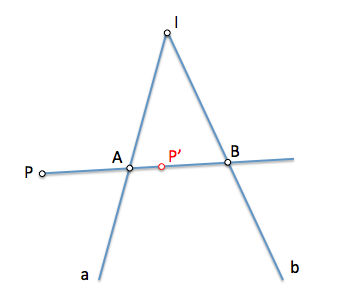We will define polar point P with respect to the straight lines “to” and “b” to the locus of the infinite points as P’ It separated harmoniously to the points of intersection, And the B, of the straight lines passing through P with “to” and “b”.

Point P’ You can get through a full cuadrivertice. We see when making the straight line construction “p” passing P’ and for its I intersection “to” and “b” It meets the conditions of this locus, It would be the diagonal of a cuadrivertice in which the point P and point I they are diagonal points.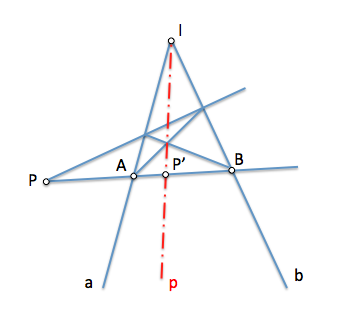• To the point P We will call you The straight p Polo
• The straight p We will call him polar p, or Polar point P

Points P and P’ they are conjugated with respect to the straight lines to and b. All points of the straight p are conjugates with respect to point P. When searching for polar with respect to any of them you must pass through P.